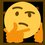# Proof of Conservation of Momentum

By Newton's Third Law of Motion,

$\mathbf{F}_{12}=-\mathbf{F}_{21}$

By Newton's Second Law of Motion,

\begin{aligned} \mathbf{F}_{12}&=-\mathbf{F}_{21}\\ \dot{\mathbf{p}}_{1}&=-\dot{\mathbf{p}}_{2}\\ \int_{t_1}^{t_2}\dot{\mathbf{p}}_{1}\,\mathrm{d}t&=-\int_{t_1}^{t_2}\dot{\mathbf{p}}_{2}\,\mathrm{d}t\\ \Delta\mathbf{p}_{1}&=-\Delta\mathbf{p}_{2}\\ \Delta(m\mathbf{v}_{1})&=-\Delta(m_2\mathbf{v}_{2})\\ m_1\Delta(\mathbf{v}_{1})&=-m_2\Delta(\mathbf{v}_{2})\\ m_1(\mathbf{v}_{1}-\mathbf{u}_{1})&=-m_2(\mathbf{v}_{2}-\mathbf{u}_{2})\\ m_1\mathbf{v}_{1}-m_1\mathbf{u}_{1}&=m_2\mathbf{u}_{21}-m_2\mathbf{v}_{2}\\ m_1\mathbf{v}_{1}+m_2\mathbf{v}_{2}&=m_2\mathbf{u}_{2}+m_1\mathbf{u}_{1}\\ \sum\mathbf{p}_{f}&=\sum\mathbf{p}_{i}\quad\blacksquare \end{aligned}Note by Gandoff Tan
1 year, 2 months ago

This discussion board is a place to discuss our Daily Challenges and the math and science related to those challenges. Explanations are more than just a solution — they should explain the steps and thinking strategies that you used to obtain the solution. Comments should further the discussion of math and science.

When posting on Brilliant:

• Use the emojis to react to an explanation, whether you're congratulating a job well done , or just really confused .
• Ask specific questions about the challenge or the steps in somebody's explanation. Well-posed questions can add a lot to the discussion, but posting "I don't understand!" doesn't help anyone.
• Try to contribute something new to the discussion, whether it is an extension, generalization or other idea related to the challenge.
• Stay on topic — we're all here to learn more about math and science, not to hear about your favorite get-rich-quick scheme or current world events.

MarkdownAppears as
*italics* or _italics_ italics
**bold** or __bold__ bold
- bulleted- list
• bulleted
• list
1. numbered2. list
1. numbered
2. list
Note: you must add a full line of space before and after lists for them to show up correctly
paragraph 1paragraph 2

paragraph 1

paragraph 2

[example link](https://brilliant.org)example link
> This is a quote
This is a quote
    # I indented these lines
# 4 spaces, and now they show
# up as a code block.

print "hello world"
# I indented these lines
# 4 spaces, and now they show
# up as a code block.

print "hello world"
MathAppears as
Remember to wrap math in $$ ... $$ or $ ... $ to ensure proper formatting.
2 \times 3 $2 \times 3$
2^{34} $2^{34}$
a_{i-1} $a_{i-1}$
\frac{2}{3} $\frac{2}{3}$
\sqrt{2} $\sqrt{2}$
\sum_{i=1}^3 $\sum_{i=1}^3$
\sin \theta $\sin \theta$
\boxed{123} $\boxed{123}$

## Comments

Sort by:

Top Newest

What happens when there are $3$ objects?

Staff - 1 year, 2 months ago

Log in to reply

Alright, let's give this a try:

Let's say we have a bunch of masses, in a system with only internal forces. where mass $m_i$ exerts a force on $m_j$ denoted by $F_{ij}$. Thus, the net force is a sum of all the internal forces:

$F_{net} \ = \ \displaystyle\sum_{i, \ j} \ F_{ij}$

By Newton's Third Law, we will have $F_{ij} \ = \ -F_{ji}$ (if $m_i$ exterts $F_{ij}$ on $m_i$, then it follows that $m_j$ exerts an equal an opposite force on $m_i$. We also know that:

$F_{net} \ = \ \frac{d p_{total}}{dt}$

Thus, we can write our sum as a bunch of $F_{ij} \ + \ F_{ji} \ = \ F_{ij} \ - \ F_{ij} \ = \ 0$ terms, which just equates to $0$, giving us:

$F_{net} \ = \ \frac{d p_{total}}{dt} \ = \ 0$

This implies that total momentum does not change with time, thus momentum is conserved when there are only internal forces acting on the system.

I probably missed a lot of nuances, how did I do @Josh Silverman? :)

- 1 year, 2 months ago

Log in to reply

I think these aren't proves, but only a verification of the law for a particular case.

- 6 months, 3 weeks ago

Log in to reply

It looks like a nose.

- 1 year, 2 months ago

Log in to reply

Yes really 😂 But good proof

- 8 months, 3 weeks ago

Log in to reply

lol

- 5 months ago

Log in to reply

×

Problem Loading...

Note Loading...

Set Loading...